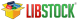`Conversions Library  mikroC PRO for PIC32 Libraries > Miscellaneous Libraries > ` `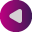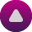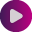`

## Conversions Library

The mikroC PRO for PIC32 Conversions Library provides routines for numerals to strings and BCD/decimal conversions.

### Library Dependency Tree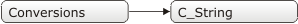### Library Routines

You can get text representation of numerical value by passing it to one of the following routines:

The following functions convert decimal values to BCD and vice versa:

### ByteToStr

Prototype void ByteToStr(unsigned short input, char *output); Converts input byte to a string. The output string has fixed width of 4 characters including null character at the end (string termination). The output string is right justified and remaining positions on the left (if any) are filled with blanks. `input:` byte to be converted `output:` destination string Nothing. Destination string should be at least 4 characters in length. ```unsigned short t = 24; char txt; ... ByteToStr(t, txt); // txt is " 24" (one blank here)``` None.

### ShortToStr

Prototype void ShortToStr(short input, char *output); Converts input signed short number to a string. The output string has fixed width of 5 characters including null character at the end (string termination). The output string is right justified and remaining positions on the left (if any) are filled with blanks. `input:` signed short number to be converted `output:` destination string Nothing. Destination string should be at least 5 characters in length. ```short t = -24; char txt; ... ShortToStr(t, txt); // txt is " -24" (one blank here)``` None.

### WordToStr

Prototype void WordToStr(unsigned input, char *output); Converts input word to a string. The output string has fixed width of 6 characters including null character at the end (string termination). The output string is right justified and the remaining positions on the left (if any) are filled with blanks. `input:` word to be converted `output:` destination string Nothing. Destination string should be at least 6 characters in length. ```unsigned t = 437; char txt; ... WordToStr(t, txt); // txt is " 437" (two blanks here)``` None.

### IntToStr

Prototype void IntToStr(int input, char *output); Converts input signed integer number to a string. The output string has fixed width of 7 characters including null character at the end (string termination). The output string is right justified and the remaining positions on the left (if any) are filled with blanks. `input:` signed integer number to be converted `output:` destination string Nothing. Destination string should be at least 7 characters in length. ```int j = -4220; char txt; ... IntToStr(j, txt); // txt is " -4220" (one blank here)``` None.

### LongToStr

Prototype void LongToStr(long input, char *output); Converts input signed long integer number to a string. The output string has fixed width of 12 characters including null character at the end (string termination). The output string is right justified and the remaining positions on the left (if any) are filled with blanks. `input:` signed long integer number to be converted `output:` destination string Nothing. Destination string should be at least 12 characters in length. ```long jj = -3700000; char txt; ... LongToStr(jj, txt); // txt is " -3700000" (three blanks here)``` None.

### LongWordToStr

Prototype void LongWordToStr(unsigned long input, char *output); Converts input unsigned long integer number to a string. The output string has fixed width of 11 characters including null character at the end (string termination). The output string is right justified and the remaining positions on the left (if any) are filled with blanks. `input:` unsigned long integer number to be converted `output:` destination string Nothing. Destination string should be at least 11 characters in length. ```unsigned long jj = 3700000; char txt; ... LongWordToStr(jj, txt); // txt is " 3700000" (three blanks here)``` None.

### LongLongUnsignedToStr

Prototype void LongLongUnsignedToStr(unsigned long long input, char *output); Converts input unsigned long long integer number to a string. The output string has fixed width of 21 characters including null character at the end (string termination). The output string is right justified and the remaining positions on the left (if any) are filled with blanks. `input:` unsigned long long integer number to be converted `output:` destination string Nothing. Destination string should be at least 11 characters in length. ```unsigned long long jj = 3700000; char txt; ... LongLongUnsignedToStr(jj, txt); // txt is " 3700000" (13 blanks here)``` None.

### LongLongSignedToStr

Prototype void LongLongSignedToStr(long long input, char *output); Converts input long long integer number to a string. The output string has fixed width of 21 characters including null character at the end (string termination). The output string is right justified and the remaining positions on the left (if any) are filled with blanks. `input:` long long integer number to be converted `output:` destination string Nothing. Destination string should be at least 11 characters in length. ```long long jj = -3700000; char txt; ... LongLongSignedToStr(jj, txt); // txt is " -3700000" (12 blanks here)``` None.

### FloatToStr

Prototype unsigned char FloatToStr(float fnum, unsigned char *str); Converts a floating point number to a string. The output string is left justified and null terminated after the last digit. `fnum:` floating point number to be converted `str:` destination string 3 if input number is NaN 2 if input number is -INF 1 if input number is +INF 0 if conversion was successful Destination string should be at least 14 characters in length. ```float ff1 = -374.2; float ff2 = 123.456789; float ff3 = 0.000001234; char txt; ... FloatToStr(ff1, txt); // txt is "-374.2" FloatToStr(ff2, txt); // txt is "123.4567" FloatToStr(ff3, txt); // txt is "1.234e-6" ``` Given floating point number will be truncated to 7 most significant digits before conversion.

### LongDoubleToStr

Prototype unsigned char LongDoubleToStr(long double dnum, unsigned char *str); Converts a long double floating point number to a string. The output string is left justified and null terminated after the last digit. `fnum:` long double floating point number to be converted `str:` destination string 3 if input number is NaN 2 if input number is -INF 1 if input number is +INF 0 if conversion was successful Destination string should be at least 23 characters in length. ```long double ff1 = -374.2; long double ff2 = 123.456789; long double ff3 = 0.000001234; char txt; ... LongDoubleToStr(ff1, txt); // txt is "-374.2" LongDoubleToStr(ff2, txt); // txt is "123.4567" LongDoubleToStr(ff3, txt); // txt is "1.234e-6" ``` None.

### WordToStrWithZeros

Prototype void WordToStrWithZeros(unsigned int input, char *output); Converts input word to a string. The output string has fixed width of 6 characters including null character at the end (string termination). The output string is right justified and remaining positions on the left (if any) are filled with zeros. `input:` unsigned integer to be converted `output:` destination string Nothing. Destination string should be at least 6 characters in length. ```unsigned short t = 437; char txt; ... WordToStrWithZeros(t, txt); // txt is "0437" (one zero here)``` None.

### IntToStrWithZeros

Prototype void IntToStrWithZeros(int input, char *output); Converts input integer to a string. The output string has fixed width of 7 characters including null character at the end (string termination). The output string is right justified and remaining positions on the left (if any) are filled with zeros. `input:` integer number to be converted `output:` destination string Nothing. Destination string should be at least 7 characters in length. ```short t = -3276; char txt; ... IntToStrWithZeros(t, txt); // txt is "-03276" (one zero here)``` None.

### LongWordToStrWithZeros

Prototype void LongWordToStrWithZeros(unsigned long input, char *output); Converts input longword to a string. The output string has fixed width of 11 characters including null character at the end (string termination). The output string is right justified and the remaining positions on the left (if any) are filled with zeros. `input:` unsigned long number to be converted `output:` destination string Nothing. Destination string should be at least 11 characters in length. ```unsigned t = 12345678; char txt; ... LongWordToStrWithZeros(t, txt); // txt is "0012345678" (two zeros)``` None.

### LongIntToStrWithZeros

Prototype void LongIntToStrWithZeros(long input, char *output); Converts input signed long integer number to a string. The output string has fixed width of 12 characters including null character at the end (string termination). The output string is right justified and the remaining positions on the left (if any) are filled with zeros. `input:` signed long number to be converted `output:` destination string Nothing. Destination string should be at least 12 characters in length. ```int j = -12345678; char txt; ... LongIntToStrWithZeros(j, txt); // txt is "-0012345678" (one zero here)``` None.

### LongLongUnsignedToStrWithZeros

Prototype void LongLongUnsignedToStrWithZeros(unsigned long long input, char *output); Converts input unsigned long long integer number to a string. The output string has fixed width of 21 characters including null character at the end (string termination). The output string is right justified and the remaining positions on the left (if any) are filled with zeros. `input:` unsigned long long number to be converted `output:` destination string Nothing. Destination string should be at least 12 characters in length. ```unsigned long long j = 1234567890; char txt; ... LongLongUnsignedToStrWithZeros(j, txt); // txt is "00000000001234567890" (ten zeros here)``` None.

### LongLongSignedToStrWithZeros

Prototype void LongLongSignedToStrWithZeros(long long input, char *output); Converts input long long integer number to a string. The output string has fixed width of 21 characters including null character at the end (string termination). The output string is right justified and the remaining positions on the left (if any) are filled with zeros. `input:` long long number to be converted `output:` destination string Nothing. Destination string should be at least 12 characters in length. ```unsigned long long j = -1234567890; char txt; ... LongLongUnsignedToStrWithZeros(j, txt); // txt is "-0000000001234567890" (nine zeros here)``` None.

### ByteToHex

Prototype void ByteToHex(char input, char *output); Converts input number to a string containing the number's hexadecimal representation. The output string has fixed width of 3 characters including null character at the end (string termination). `input:` byte to be converted `output:` destination string Nothing. Destination string should be at least 3 characters in length. ```unsigned short t = 2; char txt; ... ByteToHex(t, txt); // txt is "02"``` None.

### ShortToHex

Prototype void ShortToHex(unsigned short input, char *output); Converts input number to a string containing the number's hexadecimal representation. The output string has fixed width of 3 characters including null character at the end (string termination). `input:` signed short number to be converted `output:` destination string Nothing. Destination string should be at least 3 characters in length. ```short t = -100; char txt; ... ShortToHex(t, txt); // txt is "9C"``` None.

### WordToHex

Prototype void WordToHex(unsigned input, char *output); Converts input number to a string containing the number's hexadecimal representation. The output string has fixed width of 5 characters including null character at the end (string termination). `input:` unsigned integer to be converted `output:` destination string Nothing. Destination string should be at least 5 characters in length. ```unsigned t = 1111; char txt; ... WordToHex(t, txt); // txt is "0457"``` None.

### IntToHex

Prototype void IntToHex(int input, char *output); Converts input number to a string containing the number's hexadecimal representation. The output string has fixed width of 5 characters including null character at the end (string termination). `input:` signed integer number to be converted `output:` destination string Nothing. Destination string should be at least 5 characters in length. ```int j = -32768; char txt; ... IntToHex(j, txt); // txt is "8000"``` None.

### LongWordToHex

Prototype void LongWordToHex(unsigned long input, char *output); Converts input number to a string containing the number's hexadecimal representation. The output string has fixed width of 9 characters including null character at the end (string termination). `input:` unsigned long integer number to be converted `output:` destination string Nothing. Destination string should be at least 9 characters in length. ```unsigned long jj = 65535; char txt; ... LongWordToHex(jj, txt); // txt is "0000FFFF"``` None.

### LongIntToHex

Prototype void LongIntToHex(long int input, char *output); Converts input number to a string containing the number's hexadecimal representation. The output string has fixed width of 9 characters including null character at the end (string termination). `input:` signed long integer number to be converted `output:` destination string Nothing. Destination string should be at least 9 characters in length. ```long int jj = -2147483648; char txt; ... LongIntToHex(jj, txt); // txt is "80000000"``` None.

### LongLongUnsignedToHex

Prototype void LongLongUnsignedToHex(unsigned long long input, char *output); Converts input number to a string containing the number's hexadecimal representation. The output string has fixed width of 17 characters including null character at the end (string termination). `input:` unsigned long long integer number to be converted `output:` destination string Nothing. Destination string should be at least 17 characters in length. ```unsigned long long jj = 4294967295; char txt; ... LongLongUnsignedToHex(jj, txt); // txt is "00000000FFFFFFFF"``` None.

### LongLongSignedToHex

Prototype void LongLongSignedToHex(long long input, char *output); Converts input number to a string containing the number's hexadecimal representation. The output string has fixed width of 17 characters including null character at the end (string termination). `input:` long long integer number to be converted `output:` destination string Nothing. Destination string should be at least 17 characters in length. ```long long jj = -4294967295; char txt; ... LongLongSignedToHex(jj, txt); // txt is "-0000000FFFFFFFF"``` None.

### Dec2Bcd

Prototype unsigned short Dec2Bcd(unsigned short decnum); Converts input unsigned short integer number to its appropriate BCD representation. `decnum:` unsigned short integer number to be converted Converted BCD value. Nothing. ```unsigned short a, b; ... a = 22; b = Dec2Bcd(a); // b equals 34``` None.

### Bcd2Dec

Prototype unsigned short Bcd2Dec(unsigned short bcdnum); Converts 8-bit BCD numeral to its decimal equivalent. `bcdnum:` 8-bit BCD numeral to be converted Converted decimal value. Nothing. ```unsigned short a, b; ... a = 34; b = Bcd2Dec(22); // b equals 22``` None.

### Dec2Bcd16

Prototype unsigned Dec2Bcd16(unsigned decnum); Converts unsigned 16-bit decimal value to its BCD equivalent. `decnum` unsigned 16-bit decimal number to be converted Converted BCD value. Nothing. ```unsigned a, b; ... a = 2345; b = Dec2Bcd16(a); // b equals 9029``` None.

### Bcd2Dec16

Prototype unsigned Bcd2Dec16(unsigned bcdnum); Converts 16-bit BCD numeral to its decimal equivalent. `bcdnum:` 16-bit BCD numeral to be converted Converted decimal value. Nothing. ```unsigned a, b; ... a = 0x1234; // a equals 4660 b = Bcd2Dec16(a); // b equals 1234``` None.

### Rtrim

Prototype char *Rtrim(char *string); Trims the trailing spaces from array given with *`string` `string`: array to be trimmed. The function returns the address of the first non-space character. Nothing. ```char *res; res = Rtrim(" mikroe"); // trims the trailing spaces and returns the address of the first non-space character``` None.

### Ltrim

Prototype char *Ltrim(char *string); 66 Trims the leading spaces from array given with *`string` `string`: array to be trimmed. The function returns the address of the first non-space character. Nothing. ```char *res; res = Ltrim(" mikroe"); // trims the leading spaces and returns the address of the first non-space character``` None.
Copyright (c) 2002-2012 mikroElektronika. All rights reserved.
What do you think about this topic ? Send us feedback!
Want more examples and libraries?
Find them on